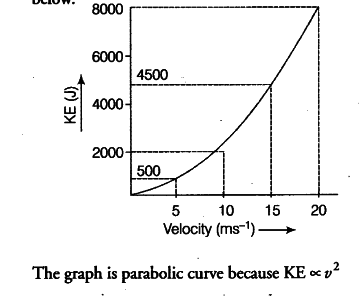# Find the KE of the boy of 40 kg

A car is moving with uniform velocities; 18km/h,36km/h,54km/h and72km/h at some intervals. Find the KE of the boy of 40 kg sitting in the car at these velocities. Draw a graph between the KE and the velocities. Also, find the nature of the curve.

At { v }_{ 1 } = 18 km/h = 18 x 1000/3600 = 5 m/s
KE = 1/2 m\${{v1}^{2}} = 1/2 x 40 x 5 x 5 = 500J At { v }{ 2 } = 36km/h =10m/s KE = 1/2 m{{v2}^{2}} = 1/2 x 40 x 10 x 10 = 2000J At { v }{ 3 } = 54 km/h = 15 m/s KE = 1/2 m{{v3}^{2}} = 1/2 x 40 x 15 x 15 = 4500J At { v }_{ 4 } = 72 km/h =20m/s KE = 1/2 m{{v4}^{2}}\$ = 1/2 x 40 x 20 x 20 = 8000J
The graph between the KE and the velocities is shown below: